Home
Hostname: page-component-6c8bd87754-hvdfp Total loading time: 0.202 Render date: 2022-01-18T14:06:41.843Z Has data issue: true Feature Flags: { "shouldUseShareProductTool": true, "shouldUseHypothesis": true, "isUnsiloEnabled": true, "metricsAbstractViews": false, "figures": true, "newCiteModal": false, "newCitedByModal": true, "newEcommerce": true, "newUsageEvents": true }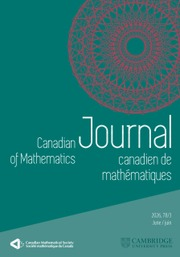Canadian Journal of Mathematics

Symplectic Degenerate Flag Varieties

Published online by Cambridge University Press:  20 November 2018

Abstract

HTML view is not available for this content. However, as you have access to this content, a full PDF is available via the ‘Save PDF’ action button.

A simple finite dimensional module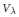${{V}_{\lambda }}$ of a simple complex algebraic group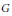$G$ is naturally endowed with a filtration induced by the PBW-filtration of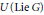$U\,(\text{Lie}\,G)$ . The associated graded space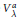$\text{V}_{\text{ }\!\!\lambda\!\!\text{ }}^{a}$ is a module for the group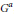${{G}^{a}}$ , which can be roughly described as a semi-direct product of a Borel subgroup of$G$ and a large commutative unipotent group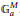$\mathbb{G}_{a}^{M}$ . In analogy to the flag variety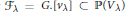${{\mathcal{F}}_{\lambda }}\,=\,G.[{{v}_{\lambda }}]\,\,\subset \,\,\mathbb{P}({{V}_{\lambda }})$ , we call the closure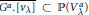$\overline{{{G}^{a}}\,.\,[{{v}_{\text{ }\!\!\lambda\!\!\text{ }}}]}\,\,\subset \,\,\mathbb{P}\,(V_{\text{ }\!\!\lambda\!\!\text{ }}^{a})$ of the${{G}^{a}}$ -orbit through the highest weight line the degenerate flag variety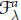$\mathcal{F}_{\text{ }\!\!\lambda\!\!\text{ }}^{a}$ . In general this is a singular variety, but we conjecture that it has many nice properties similar to that of Schubert varieties. In this paper we consider the case of$G$ being the symplectic group. The symplectic case is important for the conjecture because it is the first known case where, even for fundamental weights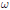$\omega$ , the varieties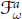$\mathcal{F}_{\text{ }\!\!\omega\!\!\text{ }}^{a}$ differ from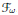${{\mathcal{F}}_{\text{ }\!\!\omega\!\!\text{ }}}$ . We give an explicit construction of the varieties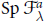$\text{Sp}\,\mathcal{F}_{\text{ }\!\!\lambda\!\!\text{ }}^{a}$ and construct desingularizations, similar to the Bott–Samelson resolutions in the classical case. We prove that$\text{Sp}\,\mathcal{F}_{\text{ }\!\!\lambda\!\!\text{ }}^{a}$ are normal locally complete intersections with terminal and rational singularities. We also show that these varieties are Frobenius split. Using the above mentioned results, we prove an analogue of the Borel–Weil theorem and obtain a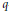$q$ -character formula for the characters of irreducible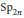$\text{S}{{\text{p}}_{2\pi }}$ -modules via the Atiyah–Bott–Lefschetz fixed points formula.

Type
Research Article
Information
Canadian Journal of Mathematics , 01 December 2014 , pp. 1250 - 1286

References

[A] Arzhantsev, I., Flag varieties as equivariant compactifications of Gna. Proc. Amer. Math. Soc. 139(2011), 783–786.http://dx.doi.org/10.1090/S0002-9939-2010-10723-2 Google Scholar
[AS] Arzhantsev, I. and Sharoiko, E., Hassett–Tschinkel correspondence: modality and projective hypersurfaces. arxiv:0912.1474.Google Scholar
[AB] Atiyah, M. F. and Bott, R., A Lefschetz fixed point formula for elliptic differential operators. Bull. Amer. Math. Soc. 72(1966), 245–250.http://dx.doi.org/10.1090/S0002-9904-1966-11483-0 Google Scholar
[E] Elkik, R., Rationalité des singularitécanoniques. Invent. Math. 64(1981), 1–6.http://dx.doi.org/10.1007/BF01393930 Google Scholar
[Fe1] Feigin, E., GMa degeneration of flag varieties. Selecta Math. 18(2012), 513–537.http://dx.doi.org/10.1007/s00029-011-0084-9 Google Scholar
[Fe2] Feigin, E., Degenerate flag varieties and the median Genocchi numbers. Math. Res. Lett. 18(2011), 1–16 http://dx.doi.org/10.4310/MRL.2011.v18.n6.a8 Google Scholar
[FF] Feigin, E. and Finkelberg, M., Degenerate flag varieties of type A: Frobenius splitting and BW theorem. Math. Z., to appear; arxiv:1103.1491.http://dx.doi.org/10.1007/s00209-012-1122-9 CrossRefGoogle Scholar
[F] Flenner, H., Rationale quasihomogene Singularitäten. Arch. Math. (Basel) 36(1981), 35–44.http://dx.doi.org/10.1007/BF01223666 Google Scholar
[FH] Fulton, W. and Harris, J., Representation theory. A first course. Graduate Texts in Math., Readings in Mathematics 129, New York, 1991.Google Scholar
[FFL1] Feigin, E., Fourier, G., and Littelmann, P., PBW filtration and bases for irreducible modules in type An. Transformation Groups 16(2011), 71–89.http://dx.doi.org/10.1007/s00031-010-9115-4 Google Scholar
[FFL2] Feigin, E., Fourier, G., and Littelmann, P. PBW filtration and bases for symplectic Lie algebras. Int. Math. Res. Not. 2011, 5760–5784.Google Scholar
[EGA] Grothendieck, A. and Dieudonné, J., Éléments de géométrie algébrique. IV. Étude locale des schémas et des morphismes de schémas. II. Inst. Hautes Études Sci. Publ. Math. 24, 1965.Google Scholar
[H] Hartshorne, R., Algebraic Geometry. Graduate Texts in Math. 52, Springer-Verlag, NewYork–Heidelberg, 1977.CrossRefGoogle Scholar
[HT] Hassett, B. and Tschinkel, Yu., Geometry of equivariant compactifications of Gna. Int. Math. Res. Not. 20(1999), 1211–1230.Google Scholar
[J] Jantzen, J. C., Representations of algebraic groups. Second edition. Math. Surveys Monogr. 107. American Mathematical Society, Providence, RI, 2003.Google Scholar
[MR] Mehta, V. B. and Ramanathan, A., Frobenius splitting and cohomology vanishing for Schubert varieties. Ann. of Math. (2) 122(1985), 27–40.http://dx.doi.org/10.2307/1971368 Google Scholar
[Ra] Ramanathan, A., Schubert varieties are arithmetically Cohen_Macaulay. Invent. Math. 80(1985), 283–294.http://dx.doi.org/10.1007/BF01388607 Google Scholar
[R] Reid, M., Young person's guide to canonical singularities. In: Algebraic geometry (Proc. Summer Res. Inst., Brunswick/Maine 1985), Proc. Sympos. Pure Math. 46(1987), 345–414.Google Scholar
[T] Thomason, R. W., Une formule de Lefschetz en K-théorie équivariante algébrique. Duke Math. J. 68(1992), 447–462.http://dx.doi.org/10.1215/S0012-7094-92-06817-7 Google Scholar
[V] Vinberg, E., On some canonical bases of representation spaces of simple Lie algebras. Conference talk, Bielefeld, 2005.Google ScholarYou have Access
11
Cited by

Send article to Kindle

Note you can select to send to either the @free.kindle.com or @kindle.com variations. ‘@free.kindle.com’ emails are free but can only be sent to your device when it is connected to wi-fi. ‘@kindle.com’ emails can be delivered even when you are not connected to wi-fi, but note that service fees apply.

Find out more about the Kindle Personal Document Service.

Symplectic Degenerate Flag Varieties
Available formats
×

Send article to Dropbox

To send this article to your Dropbox account, please select one or more formats and confirm that you agree to abide by our usage policies. If this is the first time you use this feature, you will be asked to authorise Cambridge Core to connect with your <service> account. Find out more about sending content to Dropbox.

Symplectic Degenerate Flag Varieties
Available formats
×

To send this article to your Google Drive account, please select one or more formats and confirm that you agree to abide by our usage policies. If this is the first time you use this feature, you will be asked to authorise Cambridge Core to connect with your <service> account. Find out more about sending content to Google Drive.

Symplectic Degenerate Flag Varieties
Available formats
×
×